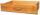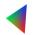# Rectangle 45

The perimeter of a rectangle is 60cm. If the length of the rectangle is 20cm.

a)find the width
b)find the area.

Result

w =  10 cm
S =  200 cm2

#### Solution:Leave us a comment of example and its solution (i.e. if it is still somewhat unclear...):

Showing 0 comments:Be the first to comment!## Next similar examples:

1. DrawerThe rectangular face of a drawer has a perimeter of 108 cm and a width of 18cm. Find the length and the area of the rectangular face of the drawer.
2. PlaygroundRectangular playground is fenced with 38 m long netting. Its width is 7 m. Calculate its length.
3. RectangleThe rectangle area is 182 dm2, its base is 14 dm. How long is the other side? Calculate its perimeter.
4. A rectangleA rectangle has an area of 36 cm2. What could the length and width of rectangle be?
5. Bathroom 2A bathroom is 2.4 meters long and 1.8 meters wide. How many square tiles 1 dm on each side are to be used to cover it?
6. Arm-legCalculate the length of the base of an isosceles triangle with a circumference 224 cm if the arm length is 68 cm.
7. Perimeter to areaCalculate the area of a circle with the perimeter 15 meters.
8. Circle - easy 2The circle has a radius 6 cm. Calculate:
9. Perimeter of squareThe square has a circumference 17cm. What is its area?
10. Bicycle wheelAfter driving 157 m bicycle wheel rotates 100 times. What is the radius of the wheel in cm?
11. 7 triangleThe triangle area is 26.7 cm2. Determine the side length l if appropriate height hl = 45.3 cm.
12. AnnulusThe radius of the larger circle is 8cm, the radius of smaller is 5cm. Calculate the contents of the annulus.
13. HotelThe hotel has a p floors each floor has i rooms from which the third are single and the others are double. Represents the number of beds in hotel.
14. SimplifySimplify the following problem and express as a decimal: 5.68-[5-(2.69+5.65-3.89) /0.5]
15. AlleyAlley measured a meters. At the beginning and end are planted poplar. How many we must plant poplars to get the distance between the poplars 15 meters?
16. ExpressionSolve for a specified variable: P=a+4b+3c, for a
17. Two machinesPerformances of two machines are in a ratio of 7:12. A machine with less power produced 406 pieces of products per shift. a) How many pieces produced per shift second machine? b) How many pieces produced two machines together for five shifts?